# Week1Lec03-Dimensional Analysis.pptx.pdf

27 May 2023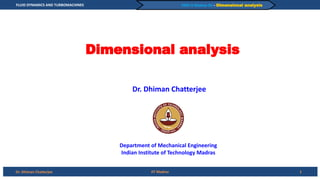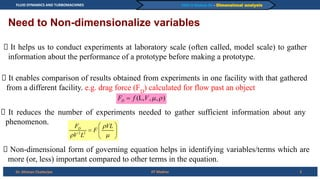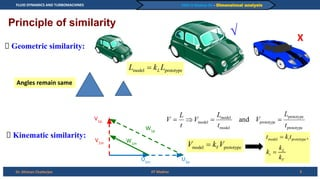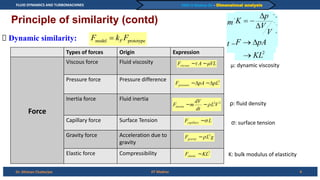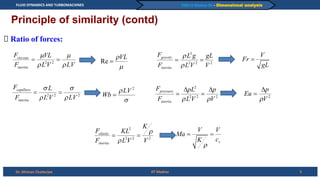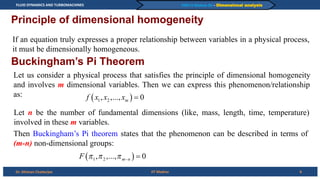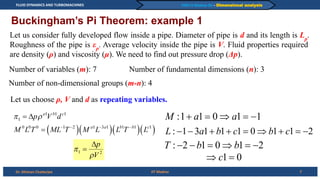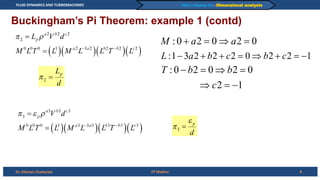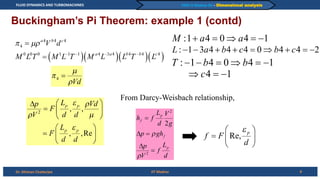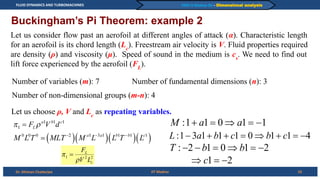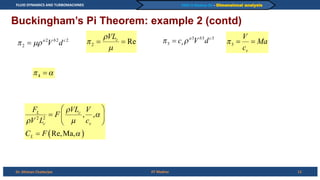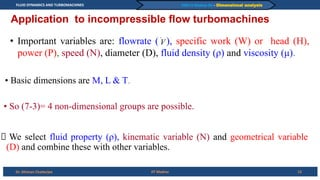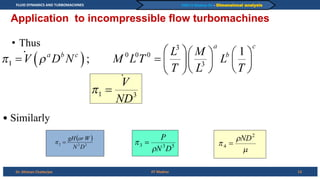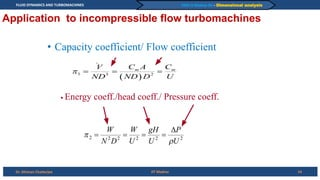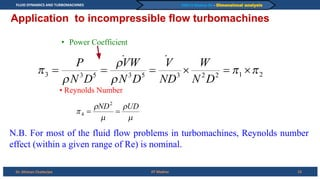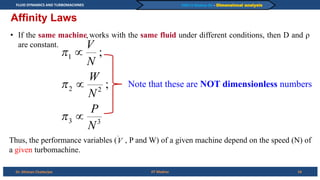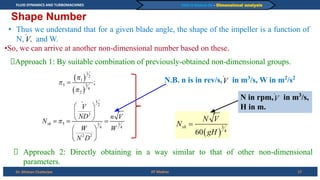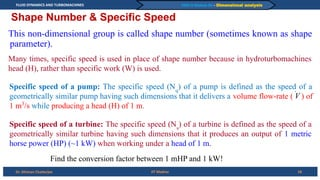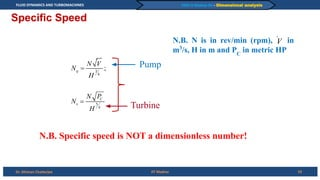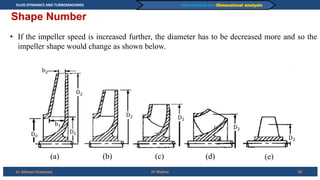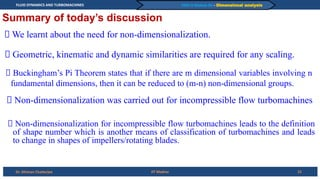1 sur 22

### Week1Lec03-Dimensional Analysis.pptx.pdf

• 1. FLUID DYNAMICS AND TURBOMACHINES Dr. Dhiman Chatterjee PART-A Module-02 – Dimensional analysis Dimensional analysis 1 IIT Madras Dr. Dhiman Chatterjee Department of Mechanical Engineering Indian Institute of Technology Madras
• 2. FLUID DYNAMICS AND TURBOMACHINES Dr. Dhiman Chatterjee PART-A Module-02 – Dimensional analysis Need to Non-dimensionalize variables IIT Madras 2 It helps us to conduct experiments at laboratory scale (often called, model scale) to gather information about the performance of a prototype before making a prototype. It enables comparison of results obtained from experiments in one facility with that gathered from a different facility. e.g. drag force (FD ) calculated for flow past an object It reduces the number of experiments needed to gather sufficient information about any phenomenon. Non-dimensional form of governing equation helps in identifying variables/terms which are more (or, less) important compared to other terms in the equation.
• 3. FLUID DYNAMICS AND TURBOMACHINES Dr. Dhiman Chatterjee PART-A Module-02 – Dimensional analysis IIT Madras 3 Principle of similarity Geometric similarity: √ X Kinematic similarity: U1m U1p V1m V1p W1m W1p Angles remain same
• 4. FLUID DYNAMICS AND TURBOMACHINES Dr. Dhiman Chatterjee PART-A Module-02 – Dimensional analysis IIT Madras 4 Principle of similarity (contd) Dynamic similarity: Types of forces Origin Expression Force Viscous force Fluid viscosity Pressure force Pressure difference Inertia force Fluid inertia Capillary force Surface Tension Gravity force Acceleration due to gravity Elastic force Compressibility μ: dynamic viscosity ρ: fluid density σ: surface tension K: bulk modulus of elasticity
• 5. FLUID DYNAMICS AND TURBOMACHINES Dr. Dhiman Chatterjee PART-A Module-02 – Dimensional analysis IIT Madras 5 Principle of similarity (contd) Ratio of forces:
• 6. FLUID DYNAMICS AND TURBOMACHINES Dr. Dhiman Chatterjee PART-A Module-02 – Dimensional analysis IIT Madras 6 Principle of dimensional homogeneity If an equation truly expresses a proper relationship between variables in a physical process, it must be dimensionally homogeneous. Buckingham’s Pi Theorem Let us consider a physical process that satisfies the principle of dimensional homogeneity and involves m dimensional variables. Then we can express this phenomenon/relationship as: Let n be the number of fundamental dimensions (like, mass, length, time, temperature) involved in these m variables. Then Buckingham’s Pi theorem states that the phenomenon can be described in terms of (m-n) non-dimensional groups:
• 7. FLUID DYNAMICS AND TURBOMACHINES Dr. Dhiman Chatterjee PART-A Module-02 – Dimensional analysis IIT Madras 7 Buckingham’s Pi Theorem: example 1 Let us consider fully developed flow inside a pipe. Diameter of pipe is d and its length is Lp . Roughness of the pipe is εp . Average velocity inside the pipe is V. Fluid properties required are density (ρ) and viscosity (μ). We need to find out pressure drop (Δp). Number of variables (m): 7 Number of fundamental dimensions (n): 3 Number of non-dimensional groups (m-n): 4 Let us choose ρ, V and d as repeating variables.
• 8. FLUID DYNAMICS AND TURBOMACHINES Dr. Dhiman Chatterjee PART-A Module-02 – Dimensional analysis IIT Madras 8 Buckingham’s Pi Theorem: example 1 (contd)
• 9. FLUID DYNAMICS AND TURBOMACHINES Dr. Dhiman Chatterjee PART-A Module-02 – Dimensional analysis IIT Madras 9 Buckingham’s Pi Theorem: example 1 (contd) From Darcy-Weisbach relationship,
• 10. FLUID DYNAMICS AND TURBOMACHINES Dr. Dhiman Chatterjee PART-A Module-02 – Dimensional analysis IIT Madras 10 Buckingham’s Pi Theorem: example 2 Let us consider flow past an aerofoil at different angles of attack (α). Characteristic length for an aerofoil is its chord length (Lc ). Freestream air velocity is V. Fluid properties required are density (ρ) and viscosity (μ). Speed of sound in the medium is cs . We need to find out lift force experienced by the aerofoil (FL ). Number of variables (m): 7 Number of fundamental dimensions (n): 3 Number of non-dimensional groups (m-n): 4 Let us choose ρ, V and Lc as repeating variables.
• 11. FLUID DYNAMICS AND TURBOMACHINES Dr. Dhiman Chatterjee PART-A Module-02 – Dimensional analysis IIT Madras 11 Buckingham’s Pi Theorem: example 2 (contd)
• 12. FLUID DYNAMICS AND TURBOMACHINES Dr. Dhiman Chatterjee PART-A Module-02 – Dimensional analysis Application to incompressible flow turbomachines • Important variables are: flowrate ( ), specific work (W) or head (H), power (P), speed (N), diameter (D), fluid density (ρ) and viscosity (μ). 12 • Basic dimensions are M, L & T. • So (7-3)= 4 non-dimensional groups are possible. We select fluid property (ρ), kinematic variable (N) and geometrical variable (D) and combine these with other variables. IIT Madras
• 13. FLUID DYNAMICS AND TURBOMACHINES Dr. Dhiman Chatterjee PART-A Module-02 – Dimensional analysis Application to incompressible flow turbomachines • Thus 13 • Similarly IIT Madras
• 14. FLUID DYNAMICS AND TURBOMACHINES Dr. Dhiman Chatterjee PART-A Module-02 – Dimensional analysis • Capacity coefficient/ Flow coefficient 14 • Energy coeff./head coeff./ Pressure coeff. IIT Madras Application to incompressible flow turbomachines
• 15. FLUID DYNAMICS AND TURBOMACHINES Dr. Dhiman Chatterjee PART-A Module-02 – Dimensional analysis • Power Coefficient 15 N.B. For most of the fluid flow problems in turbomachines, Reynolds number effect (within a given range of Re) is nominal. • Reynolds Number IIT Madras Application to incompressible flow turbomachines
• 16. FLUID DYNAMICS AND TURBOMACHINES Dr. Dhiman Chatterjee PART-A Module-02 – Dimensional analysis Affinity Laws • If the same machine works with the same fluid under different conditions, then D and ρ are constant. Note that these are NOT dimensionless numbers Thus, the performance variables ( , P and W) of a given machine depend on the speed (N) of a given turbomachine. 16 IIT Madras
• 17. FLUID DYNAMICS AND TURBOMACHINES Dr. Dhiman Chatterjee PART-A Module-02 – Dimensional analysis • Thus we understand that for a given blade angle, the shape of the impeller is a function of N, , and W. Approach 2: Directly obtaining in a way similar to that of other non-dimensional parameters. •So, we can arrive at another non-dimensional number based on these. Approach 1: By suitable combination of previously-obtained non-dimensional groups. N in rpm, in m3 /s, H in m. N.B. n is in rev/s, in m3 /s, W in m2 /s2 17 IIT Madras Shape Number
• 18. FLUID DYNAMICS AND TURBOMACHINES Dr. Dhiman Chatterjee PART-A Module-02 – Dimensional analysis Shape Number & Specific Speed This non-dimensional group is called shape number (sometimes known as shape parameter). Specific speed of a pump: The specific speed (Nq ) of a pump is defined as the speed of a geometrically similar pump having such dimensions that it delivers a volume flow-rate ( ) of 1 m3 /s while producing a head (H) of 1 m. Specific speed of a turbine: The specific speed (Ns ) of a turbine is defined as the speed of a geometrically similar turbine having such dimensions that it produces an output of 1 metric horse power (HP) (~1 kW) when working under a head of 1 m. Many times, specific speed is used in place of shape number because in hydroturbomachines head (H), rather than specific work (W) is used. Find the conversion factor between 1 mHP and 1 kW! 18 IIT Madras
• 19. FLUID DYNAMICS AND TURBOMACHINES Dr. Dhiman Chatterjee PART-A Module-02 – Dimensional analysis Specific Speed Pump Turbine N.B. Specific speed is NOT a dimensionless number! N.B. N is in rev/min (rpm), in m3 /s, H in m and PC in metric HP 19 IIT Madras
• 20. FLUID DYNAMICS AND TURBOMACHINES Dr. Dhiman Chatterjee PART-A Module-02 – Dimensional analysis • If the impeller speed is increased further, the diameter has to be decreased more and so the impeller shape would change as shown below. 20 IIT Madras Shape Number (a) (c) (b) (d) (e)
• 21. FLUID DYNAMICS AND TURBOMACHINES Dr. Dhiman Chatterjee PART-A Module-02 – Dimensional analysis IIT Madras 21 Summary of today’s discussion We learnt about the need for non-dimensionalization. Geometric, kinematic and dynamic similarities are required for any scaling. Buckingham’s Pi Theorem states that if there are m dimensional variables involving n fundamental dimensions, then it can be reduced to (m-n) non-dimensional groups. Non-dimensionalization was carried out for incompressible flow turbomachines Non-dimensionalization for incompressible flow turbomachines leads to the definition of shape number which is another means of classification of turbomachines and leads to change in shapes of impellers/rotating blades.
• 22. FLUID DYNAMICS AND TURBOMACHINES Dr. Dhiman Chatterjee PART-A Module-02 – Dimensional analysis IIT Madras 22 THANK YOU